Texas Go Math Grade 2 Lesson 3.1 Answer Key Model 4-Digit Numbers

Refer to our Texas Go Math Grade 2 Answer Key Pdf to score good marks in the exams. Test yourself by practicing the problems from Texas Go Math Grade 2 Lesson 3.1 Answer Key Model 4-Digit Numbers.

Texas Go Math Grade 2 Lesson 3.1 Answer Key Model 4-Digit Numbers

Explore

Use blocks. Draw to show your models. Write the numbers.
Aaron ___________
Tasha ___________

FOR THE TEACHER • Read the following problem. Aaron models a number with 4 hundreds, 3 tens, and 7 ones. Tasha models a different number that uses the same three digits.
Answer may vary from child to child.
Explanation:
4 hundreds, 3 tens, and 7 ones
The above given numbers are the models of Aaron.
By using the same numbers Tasha can write different model of numbers,
Example;
3 hundreds, 4 tens, and 7 ones
7 hundreds, 3 tens, and 4 ones
3 hundreds, 7 tens, and 4 ones

Math Talk
Mathematical Processes

What is the value of the digit 4 in each number you wrote?
The place value of digit 4 in each number is thousandth place.
Explanation:
There are 10 hundreds in 1 thousand.Model and Draw

There are 10 hundreds in 1 thousand.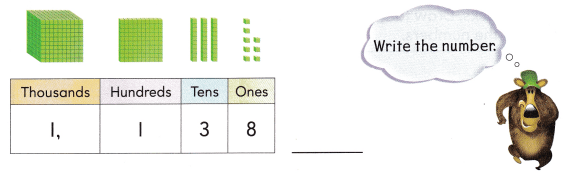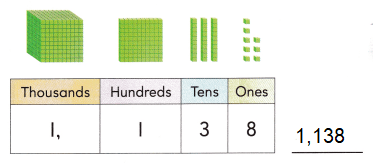Explanation:
There are 10 hundreds in 1 thousand.
There are 10 tens in 100
There are 3 tens in 30 and 8 ones in 8.
So, the number is 1,138

Share and Show

Use blocks. Complete the chart. Write the number.

Question 1.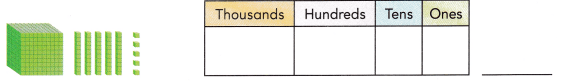Explanation:
There are 10 hundreds in 1 thousand.
There are 5 tens in 50 and 5 ones in 5.
So, the number is 1,055

Question 2.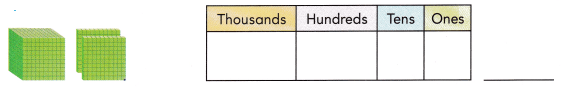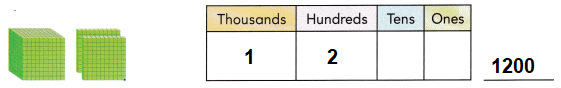Explanation:
There are 10 hundreds in 1 thousand.
There are 2 hundreds in 200
So, the number is 1,200

Question 3.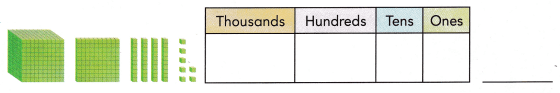Explanation:
There are 10 hundreds in 1 thousand.
There is 1 hundred in 100
There are 4 tens in 40 and 7 ones in 7.
So, the number is 1,147

Problem Solving

Use blocks. Complete the chart. Write the number.

Question 4.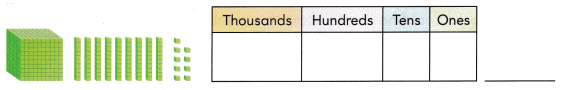Explanation:
There are 10 hundreds in 1 thousand.
There are 9 tens in 90 and 9 ones in 9.
So, the number is 1,099

Question 5.Explanation:
There are 10 hundreds in 1 thousand.
There is 1 hundred in 100
There are 2 tens in 20 and 2 ones in 2.
So, the number is 1,122

Write a number to match the clues. Use blocks if you need to.

Question 6.
H.O.T. My 4-digit number has fewer than 2 thousands. It has no hundreds, tens, or ones. What is my number?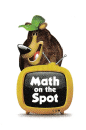Explanation:
1000 is fewer then 2000 and it has no hundreds and no ten and ones.

Question 7.
H.O.T. Multi-Step My number has 1 hundred and 1 thousand. It has double the number of tens as ones. What could my number be?
Explanation: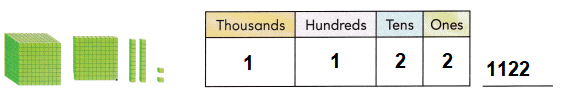Explanation:
There are 10 hundreds in 1 thousand.
There is 1 hundred in 100
There are 2 tens in 20 and 2 ones in 2.
So, the number is 1,122

Question 8.
H.O.T. Cathy wants to model the number 1,100 using only hundreds blocks. How many does she need?
She need 11 hundred blocks
Explanation:
There are 10 hundreds in 1 thousand.
There is 1 hundred in 100
So, the number is 1,100Question 9.
Connect Rob writes the number 1,150. Which list of blocks could he use to show this number?
(A) 1 thousand, 1 hundred, 5 tens, and 0 ones
(B) 1 thousand, 5 hundreds, 1 ten, and 0 ones
(C) 0 thousands, 5 hundreds, 1 ten, and 1 one
Option (A)
Explanation:
Rob has to use 10 hundreds in 1 thousand block.
1 hundred block in 100
There are 5 ten blocks in 50
So, the number is 1,150

Question 10.
Representations Bree writes a number as I thousand, 8 hundreds, 9 tens, and 7 ones. Which is another way to write her number?
(A) 1,879
(B) 1,798
(C) 1,897
Option (C)
Explanation:
In the above the number is represented with place values.
Put all the place values in the ascending order to write the number in another form.
So, the number is 1,897

Question 11.
Connect Marita’s number has the digit 7 in the hundreds place. Which of the following could be her number?
(A) 1,287
(B) 1,278
(C) 1,728
Option(C)
Explanation:
From the above options,
option C has the digit 7 in the hundreds place.

Question 12.
TEXAS Test Prep Which of these numbers is shown with the model?(A) 243
(B) 1,143
(C) 1,138
Option (B)
Explanation:
The above model,
There are 10 hundreds in 1 thousand.
There is 1 hundred in 100
There are 4 tens in 40 and 3 ones in 3.
So, the number is 1,138

TAKE HOME ACTIVITY • Have your child explain how he or she solved one of the exercises in this lesson.
It differs from child learning.
Explanation:
By bringing the concept of blocks one can easily understand the place values and write the numbers.

Texas Go Math Grade 2 Lesson 3.1 Homework and Practice Answer Key

Use blocks. Complete the chart. Write the number.

Question 1.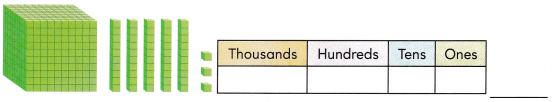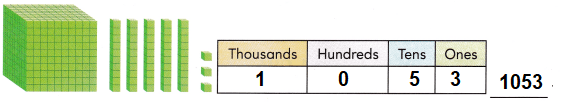Explanation:
There are 10 hundreds in 1 thousand.
There are 5 tens in 50 and 3 ones in 3.
So, the number is 1,053

Question 2.Explanation:
There are 10 hundreds in 1 thousand.
There is 1 hundred in 100
There are 7 tens in 70 and 8 ones in 8.
So, the number is 1,178

Problem Solving

Write a number to match the clues. Use blocks if you need to.

Question 3.
Ryan wants to model the number 1,200 using only hundreds blocks. How many does he need? Draw or write to explain your answer.
She need 12 hundred blocks.Explanation:
There are 10 hundreds blocks in 1 thousand.
There are 2 hundred blocks in 200.
So, the number is 1,200
He need 12 hundred blocks in all.

Question 4.
Multi-Step My number has 2 hundreds and 1 thousand. It has 4 ones and one more tens blocks than ones blocks. What is my number?
Explanation:
There are 10 hundreds in 1 thousand block.
There are 2 hundred blocks in 200
There are 1 tens block in 10 and 4 ones block in 4.
So, the number is 1,122Lesson Check

Question 5.
What is the number?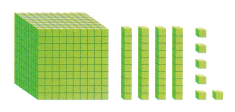(A) 146
(B) 1,046
(C) 461
Option (B)
Explanation:
There are 10 hundreds blocks in 1 thousand.
There are 4 tens block in 40 and 6 ones block in 6.
So, the number is 1,046

Question 6.
Dena models a number. Her number has a 5 in the tens place. Which of the following could be her number?
(A) 1,153
(B) 571
(C) 1,095
Option (A)
Explanation:
From the above options, option B is correct as it has 5 in tens place.
Question 7.
Shown writes a number as 1 thousand, 1 hundred, 3 tens. and 9 ones. Which is another way to write his number?
(A) 1,193
(B) 1,039
(C) 1,139
Option (C)
Explanation:
In the above the number is represented with place values.
Put all the place values in the ascending order to write the number in another form.
So, the number is 1,139

Question 8.
Brenda writes the number 1,052 on a card. Which is another way to write Brenda’s number?
(A) 1 thousand, 0 hundreds, 2 tens, and 5 ones
(B) 1 thousand, 0 hundreds, 5 tens, and 2 ones
(C) 1 thousand, 0 hundreds, 2 tens, and 5 ones